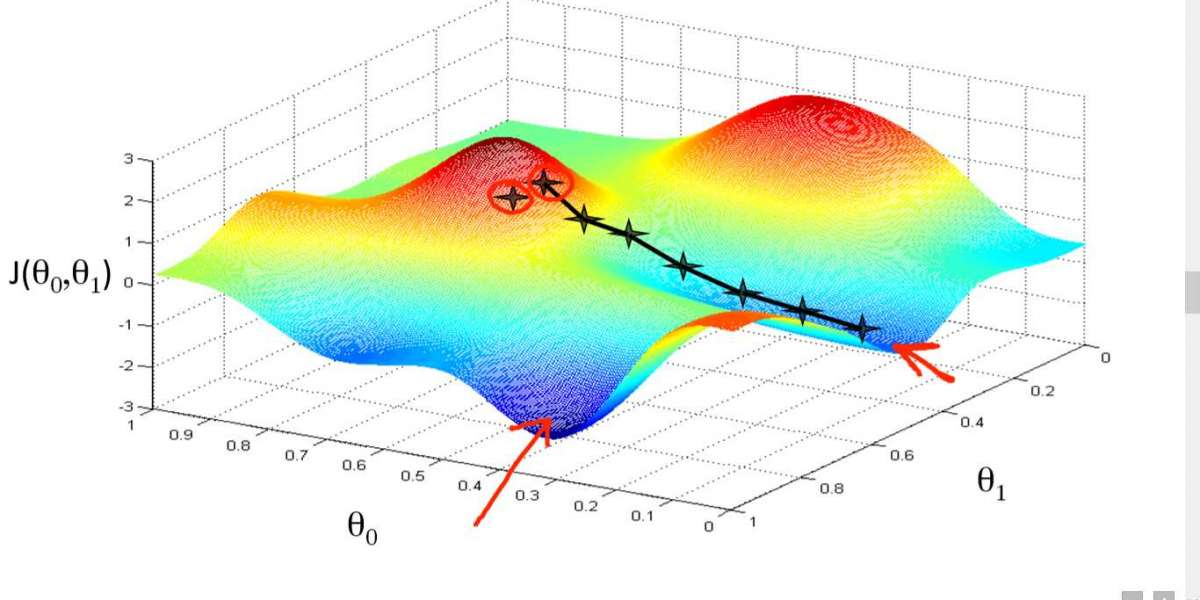## Gradient Descent : Gradient Descent is an optimization Algorithm for finding, Updating weights, Coefficients of the Algo

The three main types of Gradient Descent Algorithms are :

1. Stochastic Gradient Descent(After one training Example, the weights are updated)
2. Batch Gradient Descent(After one Epoch (after parsing through all the training examples), the weight of the model is updated at once)
3. Mini-Batch Gradient Descent(Some set of training examples(such as 32,64,128 rows of the data set are broken down and taken as training set and then update the weights of the model))

How it works?

Basic Steps :(As simple as that)

Train Data - Make Predictions - Check the Wrong Predictions (Calculate the Loss function, i.e, How many wrong predictions?) - Update the parameters of the algorithms and re-run the model again with different parameters(Updated values) and reduce the loss function - Iterate the loop again and repeat the process.

First, What does a Gradient Mean?

Gradient is a simple term used for Slope of a function.

Gradient Descent means, Finding Slope’s Descent.

To Understand this briefly,

Let us consider a Neural Network.

Consider a row of a data set.

The parameters of the training algorithm are Weights(W), Bias(b).

With a particular weight(for ex) we take 0.3, b=0.1

We get a output function(Wx+b) from a neuron = A particular value.

The output function(Wx+b) of a particular neuron is represented by below given diagram, and the black ball is at value of function at W=0.3,b=0.1.

But after training, We found that the loss function was high(Many wrong predictions). So we try to find newer weights and bias values. So we need to update the values of model parameters from W=0.3 to ??

We will find the Updated values of weights by using Gradient Descent Algorithm, which means find the slope’s descent at the same point and check whether is there further slope descent?

Black Point : W=0.3

The Gradient Descent(Derivation of loss function at that point and if there is a slope in downward direction, then we reduce that from the original weight(W=W-alpha(dL/dW), also k/a Updated Weight) and then we use this and re-run the model and find lower weights and bias parameters for the Loss functions, giving a more accurability in the model and reach the lowest loss point( referred to as Global Loss Minimum)

In the Above example, we just considered one row value of the data set at a time and update the weight and bias value and re-ran the algorithm(One Epoch). This is known as Stochastic Gradient Descent Algorithm.Advantages are Faster Learning and Easy to Implement.

Dis-Advantages : Updating the model frequently, is computationally costly affair, taking longer time to train.

Variation of Gradient Descent, Calculates error for each training example in the data set and updates the parameter values in the end, after all the examples have been evaluated at once. After a single epoch(Through all data set examples), the weights are updated once. But in case of Stochastic Gradient Descent, weights are updated for each and every training examples.